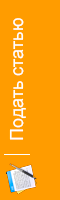Akymov V. Deep learning algorithms for classification of skin diseases // International scientific journal "Internauka". — 2018. — №9.

Отрасль науки: Технические науки

Technical Sciences

UDC 005.63:657:631.11

Student of the

National Technical University of Ukraine

“Igor Sikorsky Kyiv Polytechnic Institute”

DEEP LEARNING ALGORITHMS FOR CLASSIFICATION OF SKIN DISEASES

Summary. Skin diseases today are among the most common medical problems. The amount of these diseases is constantly growing, despite the development of medicine. Skin cancer is a common malignant neoplasm and has the second rank in the structure of cancer morbidity in Ukraine. Such diseases are diagnosed visually, beginning with clinical examinations, which can be accompanied by dermatoscopy analysis, biopsy and histopathological examination. Of particular interest is the automated classification of skin diseases (both benign and malignant) based on the image of the affected area of the body. Convolutional Neural Networks (CNN) show the potential for analyzing the category of fine-grained images.

Key words: deep learning algorithms, Convolutional Neural Networks (CNN), classification, skin diseases, fine-grained images.

Training Dataset. The training sample of data is presented by the International Society for Digital Imaging of the Skin (ISDIS) and contains 13,791 images of affected skin areas made with a dermatoscope .

Neural Network Architecture. Let's take a look at the architecture of the convolutional network, which was used to classify diseases. This network is a sequence of layers of the following types: convolutional, aggregating (or sub-sample layer) and fully connected .

Fig. 1. Architecture of a multilayer convolutional neural network

Input layer. Contains raw pixel intensities. Images have a width and height of 150px and three color channels: R, G, B.

A convolution layer. Convolution is a linear transformation of input data. Let, then the result of a two-dimensional convolution with a-sized core and a weight matrix W on the next layer is: where is a result of convolution at the l level, and is its input, i.e. the output of the whole previous layer. In other words, in order to obtain a component (i, j) of the next level, it is necessary to use the linear transformation to the square window of the previous level, i.e. scalarly multiply the pixels from this window by the convolution core .

Fig. 2. Visualization of the convolution operation

To introduce non-linearity into the model, the rectifier activation function (ReLU) is used:

Aggregating layer. It is used to reduce the number of dimensions of the input feature card by generalizing them and losing an insignificant part of information about their position. In this task, it is recommended to use the maximum element selection method: where d is the size of the sub-sample layer window.

Fully connected layer. After several passes of the image convolution and layers of the subsample, the system is rebuilt from a specific grid of pixels with high resolution to more abstract feature cards. Usually, on each next layer the amount of channels increases and number of dimensions of the image within each channel decreases. This data is combined and transferred to a conventional fully connected neural network, which is represented as follows:

Fig. 3. Visualization of a fully connected layer

As the activation function for the final layer, SoftMax is used, which converts the input vector into the probability vector of the object's belonging to the corresponding class.

Estimation of the accuracy of the obtained model. Let’s take a look at the concept of cross entropy (or Kullback-Leibler divergence), which is a measure of the divergence between two probability distributions of P and Q. Usually, it is considered that P is the true distribution, and Q is its approximation, then the Kullback-Leibler divergence serves as an estimation of the quality of approximation . In the case when P and Q are discrete random variables on a discrete set the Kullback-Leibler divergence is as follows:

where і are the actual probabilities of the result. As an error function, the cross entropy is used:

For discrete p and q, we have:

Conclusions. Thus, to summarize, automatic diagnosis of skin diseases is feasible and achievable through the usage of deep learning techniques, especially Convolutional Neural Networks (CNN). Overall accuracy of proposed model can be estimated using cross entropy which is a measure of the divergence between two probability distributions. Further, the improvement in classification model might also be achieved through the use of predefined feature selection methods.

References

1. Nikolenko, S., Kadurin, A. and Arkhangelskaya, E. (2018). Deep learning. Immersion in the world of neural networks.. 2nd ed. Saint Petersburg: Piter, p. 480.
2. Nikhil Buduma (2017). Fundamentals of Deep Learning. Designing next-generation machine intelligence algorithms. 978-1-491-92561-4. Newton: O’Reilly Media, p. 277.
3. Francois Chollet (2017). Deep Learning with Python. 978-1617294433. Shelter Island: Manning Publications, p.384.
4. Lu, L., Zheng, Y., Carneiro, G. and Yang, L. (2017). Deep Learning and Convolutional Neural Networks for Medical Image Computing. 978-3-319-42998-4 Cham: Springer International Publishing, p. 326.